Three-phase EMI filter. LTspice

AnTel

Joined Oct 30, 2020
5
Hello. Could you help me understand how to create a three-phase EMI filter? For example, I chose a full-bridge inverter with parameters P = 100kW, f_switch = 100 kHz. The noise measurement is performed using the LISN.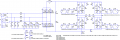The results of the simulation (calculation time 0-50ms)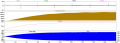In this graph, I don't like the presence of peaks for the differential and common mode noise. Could you answer, this is how it should be?
The results of the simulation (calculation time 45-50ms)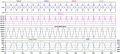Differential and common mode noise spectrum (calculation time 45-50ms)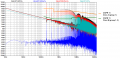Next, I want to design an input filter. If I understand correctly, the main condition for filter design is that the filter impedance should be less than the input impedance of the inverter (about 10 dB). Question: How to plot the input impedance of the inverter?
I simplified the circuit and set AC = 1 for the Vg source.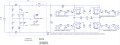The inverter input impedance graph is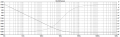I chose a filter frequency of about 100Hz. From the previous graph, I determine that at a frequency of 100Hz, the input impedance of the inverter is -21dB. This means that the impedance of the filter should be -21dB-10dB = -31dB (0.028Ω). Could you correct me if I am wrong? I think the plotted input impedance plot is wrong. Maybe I need to add a PFC in front of the inverter circuit?

Attachments

• 10.7 KB Views: 29
• 18.1 KB Views: 31

eetech00

Joined Jun 8, 2013
2,822
Hello. Could you help me understand how to create a three-phase EMI filter? For example, I chose a full-bridge inverter with parameters P = 100kW, f_switch = 100 kHz. The noise measurement is performed using the LISN.
View attachment 220998

The results of the simulation (calculation time 0-50ms)
View attachment 220999

In this graph, I don't like the presence of peaks for the differential and common mode noise. Could you answer, this is how it should be?
The results of the simulation (calculation time 45-50ms)
View attachment 221000

Differential and common mode noise spectrum (calculation time 45-50ms)
View attachment 221001

Next, I want to design an input filter. If I understand correctly, the main condition for filter design is that the filter impedance should be less than the input impedance of the inverter (about 10 dB). Question: How to plot the input impedance of the inverter?
I simplified the circuit and set AC = 1 for the Vg source.
View attachment 221002

The inverter input impedance graph is
View attachment 221003

I chose a filter frequency of about 100Hz. From the previous graph, I determine that at a frequency of 100Hz, the input impedance of the inverter is -21dB. This means that the impedance of the filter should be -21dB-10dB = -31dB (0.028Ω). Could you correct me if I am wrong? I think the plotted input impedance plot is wrong. Maybe I need to add a PFC in front of the inverter circuit?
Add some series resistance (100m ohms)to the AC voltage source "Vg".